International
Tables for
Crystallography
Volume B
Reciprocal space
Edited by U. Shmueli

International Tables for Crystallography (2010). Vol. B, ch. 1.3, pp. 66-67   | 1 | 2 |

## Section 1.3.4.2.1.8. Sections and projections

G. Bricognea

aGlobal Phasing Ltd, Sheraton House, Suites 14–16, Castle Park, Cambridge CB3 0AX, England, and LURE, Bâtiment 209D, Université Paris-Sud, 91405 Orsay, France

#### 1.3.4.2.1.8. Sections and projections

| top | pdf |

It was shown at the end of Section 1.3.2.5.8that the convolution theorem establishes, under appropriate assumptions, a duality between sectioning a smooth function (viewed as a multiplication by a δ-function in the sectioning coordinate) and projecting its transform (viewed as a convolution with the function 1 everywhere equal to 1 as a function of the projection coordinate). This duality follows from the fact thatandmaptoandto(Section 1.3.2.5.6), and from the tensor product property (Section 1.3.2.5.5).

In the case of periodic distributions, projection and section must be performed with respect to directions or subspaces which are integral with respect to the period lattice if the result is to be periodic; furthermore, projections must be performed only on the contents of one repeating unit along the direction of projection, or else the result would diverge. The same relations then hold between principal central sections and projections of the electron density and the dual principal central projections and sections of the weighted reciprocal lattice, e.g.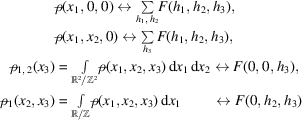etc.

When the sections are principal but not central, it suffices to use the shift property of Section 1.3.2.5.5. When the sections or projections are not principal, they can be made principal by changing to new primitive bases B andfor Λ and, respectively, the transition matrices P andto these new bases being related byin order to preserve duality. This change of basis must be such that one of these matrices (say, P) should have a given integer vector u as its first column, u being related to the line or plane defining the section or projection of interest.

The problem of constructing a matrix P given u received an erroneous solution in Volume II of International Tables (Patterson, 1959), which was subsequently corrected in 1962. Unfortunately, the solution proposed there is complicated and does not suggest a general approach to the problem. It therefore seems worthwhile to record here an effective procedure which solves this problem in any dimension n (Watson, 1970).

Letbe a primitive integral vector, i.e. g.c.d.. Then anintegral matrix P with dethaving u as its first column can be constructed by induction as follows. Forthe result is trivial. Forit can be solved by means of the Euclidean algorithm, which yieldssuch that, so that we may take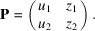Note that, ifis a solution, thenis another solution for any. For, writewithso that both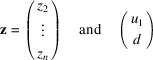are primitive. By the inductive hypothesis there is an integralmatrix V with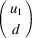as its first column, and an integralmatrix Z with z as its first column, withand.

Now puti.e.The first column of P isand its determinant is 1, QED.

The incremental step from dimensionto dimension n is the construction ofmatrix V, for which there exist infinitely many solutions labelled by an integer. Therefore, the collection of matrices P which solve the problem is labelled byarbitrary integers. This freedom can be used to adjust the shape of the basis B.

Once P has been chosen, the calculation of general sections and projections is transformed into that of principal sections and projections by the changes of coordinates:and an appeal to the tensor product property.

Booth (1945a)made use of the convolution theorem to form the Fourier coefficients of `bounded projections', which provided a compromise between 2D and 3D Fourier syntheses. If it is desired to compute the projection on the (x, y) plane of the electron density lying between the planesand, which may be written asThe transform is thengiving for coefficient: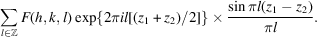### References

Booth, A. D. (1945a). Two new modifications of the Fourier method of X-ray structure analysis. Trans. Faraday Soc. 41, 434–438.
Patterson, A. L. (1959). In International Tables for X-ray Crystallography, Vol. II, pp. 9–10. Erratum, January 1962. Birmingham: Kynoch Press.
Watson, G. L. (1970). Integral Quadratic Forms. Cambridge University Press.